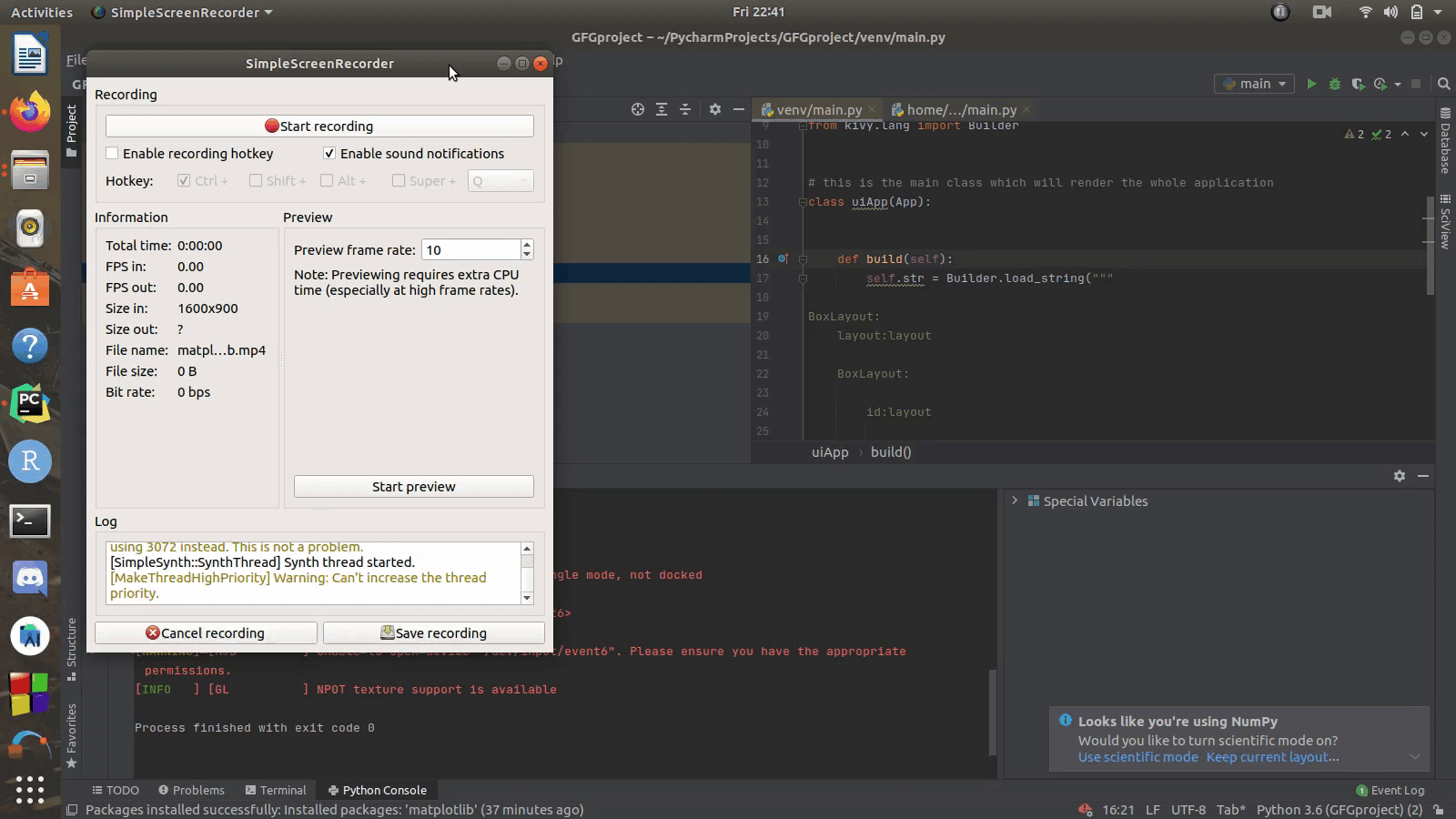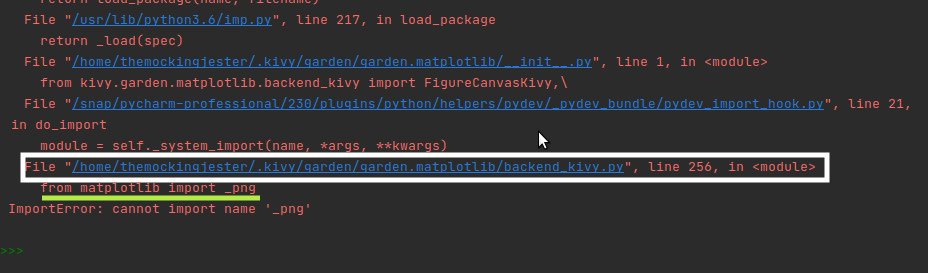# How to add Matplotlib graph in Kivy ?

• Difficulty Level : Hard
• Last Updated : 05 Apr, 2021

#### Approach:

• Import matplotlib pyplot
• Import numpy
• Import FigureCanvas KivyAgg
• Import kivy app
• Import kivy builder
• Create App class
• Return builder string
• Run an instance of the class

Below is the Implementation.

## Python3

 `# importing pyplot for graph plotting``from` `matplotlib ``import` `pyplot as plt`` ` `# importing numpy``import` `numpy as np``from` `kivy.garden.matplotlib ``import` `FigureCanvasKivyAgg`` ` `# importing kivyapp``from` `kivy.app ``import` `App`` ` `# importing kivy builder``from` `kivy.lang ``import` `Builder`` ` ` ` `# this is the main class which will ``# render the whole application``class` `uiApp(App):`` ` `    ``def` `build(``self``):``        ``self``.``str` `=` `Builder.load_string(``""" `` ` `BoxLayout:``    ``layout:layout``     ` `    ``BoxLayout:``     ` `        ``id:layout``     ` `                                ``"""``)`` ` `        ``signal ``=` `[``7``, ``89.6``, ``45.``-``56.34``]`` ` `        ``signal ``=` `np.array(signal)``         ` `        ``# this will plot the signal on graph``        ``plt.plot(signal)``         ` `        ``# setting x label``        ``plt.xlabel(``'Time(s)'``)``         ` `        ``# setting y label``        ``plt.ylabel(``'signal (norm)'``)``        ``plt.grid(``True``, color``=``'lightgray'``)``         ` `        ``# adding plot to kivy boxlayout``        ``self``.``str``.layout.add_widget(FigureCanvasKivyAgg(plt.gcf()))``        ``return` `self``.``str`` ` ` ` `# running the application``uiApp().run()`

Output:Note: When you run the below code this may throw the error given belowWhat you have to do is open the file given in the white box by clicking on file while holding ctrl key and comment line underlined by green color in that file and hit save now you will be able to run it!!

My Personal Notes arrow_drop_up